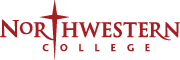# Elementary Endorsement in Mathematics

(For elementary education majors who want a subject specialty in mathematics)
Requires a minimum of 12 credits of elementary student teaching (EDU413).

### Requirements

CSC 171QR - Computer Science I
(4 credits) (NWCore option under Quantitative Reasoning) This is the first in a two-semester sequence of courses that introduces students to fundamental aspects of the field of computing; focusing on problem-solving, software design concepts and their realization as computer programs. Topics include procedural abstraction, control structures, iteration, data types and their representation. An introduction to a high-level language, for the purpose of gaining mastery of these principles, will be provided in lectures and hands-on laboratory experiences. Prerequisite: ACT math score of 20 or above (SAT 510 or above).
MAT 112QR - Calculus I
(4 credits) (NWCore option under Quantitative Reasoning) This course is a study of functions, limits, derivatives and integrals with a strong emphasis on both theory and applications. Prerequisites: C- or higher in MAT109, or an ACT math score of at least 24 (SAT 570 or above), or permission of mathematics department chair. Note: Meets four times per week.
MAT 180WI - Logic and Discrete Mathematics
(3 credits) (Writing intensive) An introduction to the language and logic of mathematical proof via topics in discrete mathematics. Topics will include logic, elementary number theory, basic set theory and methods of mathematical proof (direct proof, indirect proof, induction). Prerequisite: C- or better in MAT 112QR or permission of instructor.Note: Other topics will be chosen from counting, functions, relations, recursion and graph theory.
MAT 211 - Calculus II
(4 credits) A study of transcendental functions, techniques of integration, improper integration, sequences, series, polar coordinates and conic sections. Prerequisite: C- or better in MAT112QR or permission of instructor.Note: Meets four times per week.
MAT 317 - Linear Algebra
(3 credits) A study of the algebra of matrices and vector spaces including matrix operations, systems of equations, determinants, properties of real vector spaces, eigenvalues and eigenvectors. Prerequisite: C- or better in MAT112QR and MAT180 or permission of instructor.
MAT 411 - College Geometry
(3 credits, alternate years, consult department) A study of topics in Euclidean geometry as well as axiomatic systems, non- Euclidean geometry, and projective geometry. Prerequisite: C- or better in MAT180WI or permission of instructor.
Choose one course: 3
MAT 116QR - Statistics for the Natural and Social Sciences
(3 credits) (NWCore option under Quantitative Reasoning) This course is designed to introduce topics in probability and statistics with an emphasis on problems in the sciences. We will study discrete and continuous distributions, estimation and hypothesis testing, p-values, correlation, and simple linear regression. There will be three main sections in this course, examining descriptive statistics (the nature of data and how to summarize it), basic probability concepts (the mathematical study of uncertainty), and inferential statistics (making claims or decisions based on one or more sets of data). Prerequisite: ACT math score of 20 or above (SAT 510 or above), ALEKS placement exam score of 46 or above, concurrent enrollment in MAT100, or permission of instructor. Note: Students may receive credit for only one course among MAT116QR, MAT117QR and MAT208QR.
MAT 117QR - Applied Statistics for Management
(3 credits) (NWCore option under Quantitative Reasoning) The course is designed to study statistical methodology commonly used in business including descriptive statistics (the nature of data and how to summarize it), basic probability concepts, and inferential statistics (making claims or decisions from one or more sets of data using confidence intervals and multiple types of hypothesis testing). Prerequisite: ACT math score of 20 or above (SAT 510 or above), ALEKS placement exam score of 46 or above, concurrent enrollment in MAT100, or permission of instructor. Note: Students may receive credit for only one course among MAT 116QR, MAT 117QR and MAT 208QR.

### Total credits required: 24